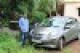# Write a Java program to convert a decimal number to binary numbers

## Introduction

In this demo I have used NetBeans IDE 8.2 for debugging purpose. But you can use any java programming language compiler as per your availability..

```import java.util.Scanner;
public class Javaexcercise {
public static void main(String args[])
{
int decimalnum, quot, i=1, j;
int bin_num[] = new int;
Scanner scan = new Scanner(System.in);

System.out.print("Enter a Decimal Number : ");
decimalnum = scan.nextInt();

quot = decimalnum;

while(quot != 0)
{
bin_num[i++] = quot%2;
quot = quot/2;
}

System.out.print("Binary number is: ");
for(j=i-1; j>0; j--)
{
System.out.print(bin_num[j]);
}
System.out.print("\n");
}
}```

# Result

### 3 thoughts on “Write a Java program to convert a decimal number to binary numbers”

1.package myfirstpackage;

import java.util.Scanner;
public class experiment {

public static void main(String[] args) {
int num, harry;
Scanner sc = new Scanner(System.in);
System.out.println(“Please Enter the Decimal Number :”);
num = sc.nextInt();

while (num>0)
{
harry = num % 2;
System.out.print(harry);
num = num/2;

}
}
}

i got the same result of Decimal to Binary Number.
I think so that it is easy code. And main thing is that i performed this code without having array in my code. Rather than, i executed the same ans using just two numbers. If there is any error, so please email me:
hamzamessageroutlock@gmail.com

2.•